# About psychonetrics

$$\textbf{psychonetrics}$$ is an R package written by Sacha Epskamp from the University of Amsterdam, which can be used for building and testing Structural Equations Models and Network Models. Its aim is to bridge the gap between the two approaches. In this tutorial we introduce how to use $$\textbf{psychonetrics}$$ for Structural Equation Modeling. We will guide you through building, fitting, modifying, and comparing models based on an easily accessible dataset.

# Installation guidelines

You can download and install it by going to https://github.com/SachaEpskamp/psychonetrics#installation, and following the instructions from there. Please note that the $$\textbf{psychonetrics}$$ package is still in its beta version, meaning that detailed aspects of the package may still be unstable or change in future versions.

# Building a Model

A structural equation model model is used to represent visually and numerically the relationships between variables in a dataset. It is a hypothesis we have about said relationships that we need to test. The variables we analyze can be either only manifest ones, i.e. variables that we can measure directly and that we have in our database, or manifest and latent. Latent variables are not (and usually cannot be) directly observed, but we hypothesize that they can be measured through manifest variables, The latter are also called indicators.

There are two types of models: measurement and structural. These models are in principle the same, except that in a measurement model the latent variables are allowed to covary freely, while in a structural model a causal relationship between these variables is assumed and tested. This tutorial will show you how to build the latter.

Let’s say we want to test our hypothesis that industrialization has an influence on democracy. Here we encounter our first problem: there is no instrument we could use to directly measure either industrialization or the state of democracy. But we have an educated hunch that we could get a good measure of industrialization by looking at GNP per capita, energy consumption per capita, and the percentage of labour force in industry. In the same way, we believe that we can measure the state of democracy by observing expert ratings on freedom of the press, the freedom of political opposition, the fairness of elections, and the effectiveness of the elected legislature. These latter variables, which we can observe, are called indicators. The underlying assumption is that there are latent variables that are a common cause for each set of indicators respectively. In our case, changes in the state of democracy will cause, - and thus can be measured through changes in - , for example, the freedom of political opposition. As such, a change in the latent variable will be related to a change in the observed variables, i.e. an increase in industrialization will be reflected by increases in GNP/capita, energy consumption, and labour force in industry.

We have data for 75 countries about the state of democracy in 1960 and 1965, and about industrialization in 1960. We hypothesize that the indicators for democracy stay the same over the years, as it is the same concept just measured at different time points. We further hypothesize that industrialization in 1960 influences democracy in 1960 and in 1965. Moreover, we hypothesize that democracy in 1960 influences democracy in 1965. The model we have in mind looks like this: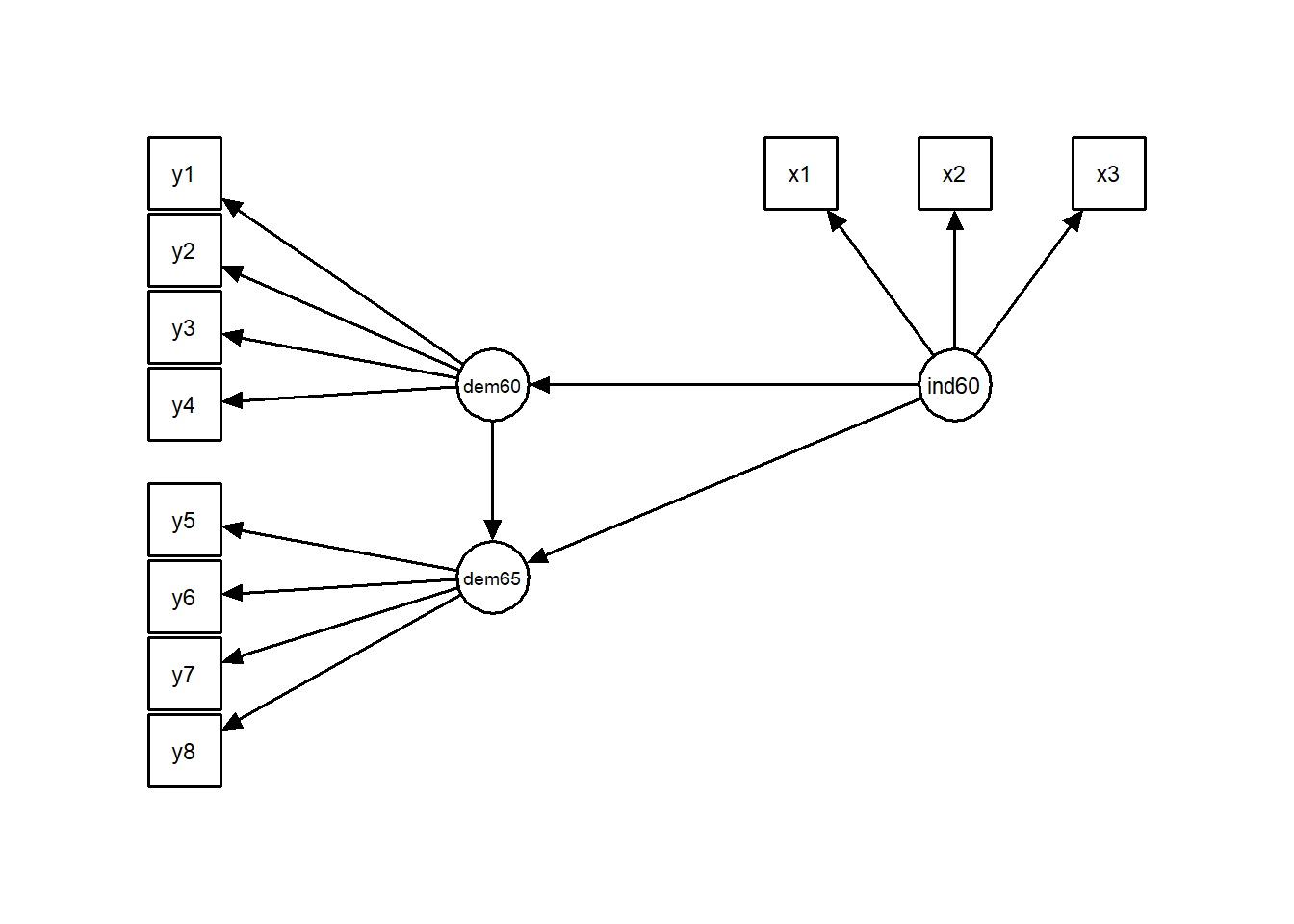Where x$$_1$$ - x$$_3$$ are the indicators for industrialization in 1960, y$$_1$$ - y$$_4$$ are the indicators for democracy in 1960, and y$$_5$$ - y$$_8$$ are the same indicators for the state of democracy, but measured in 1965.

Let’s start to assemble the building blocks for our model. First we build our democracy variable: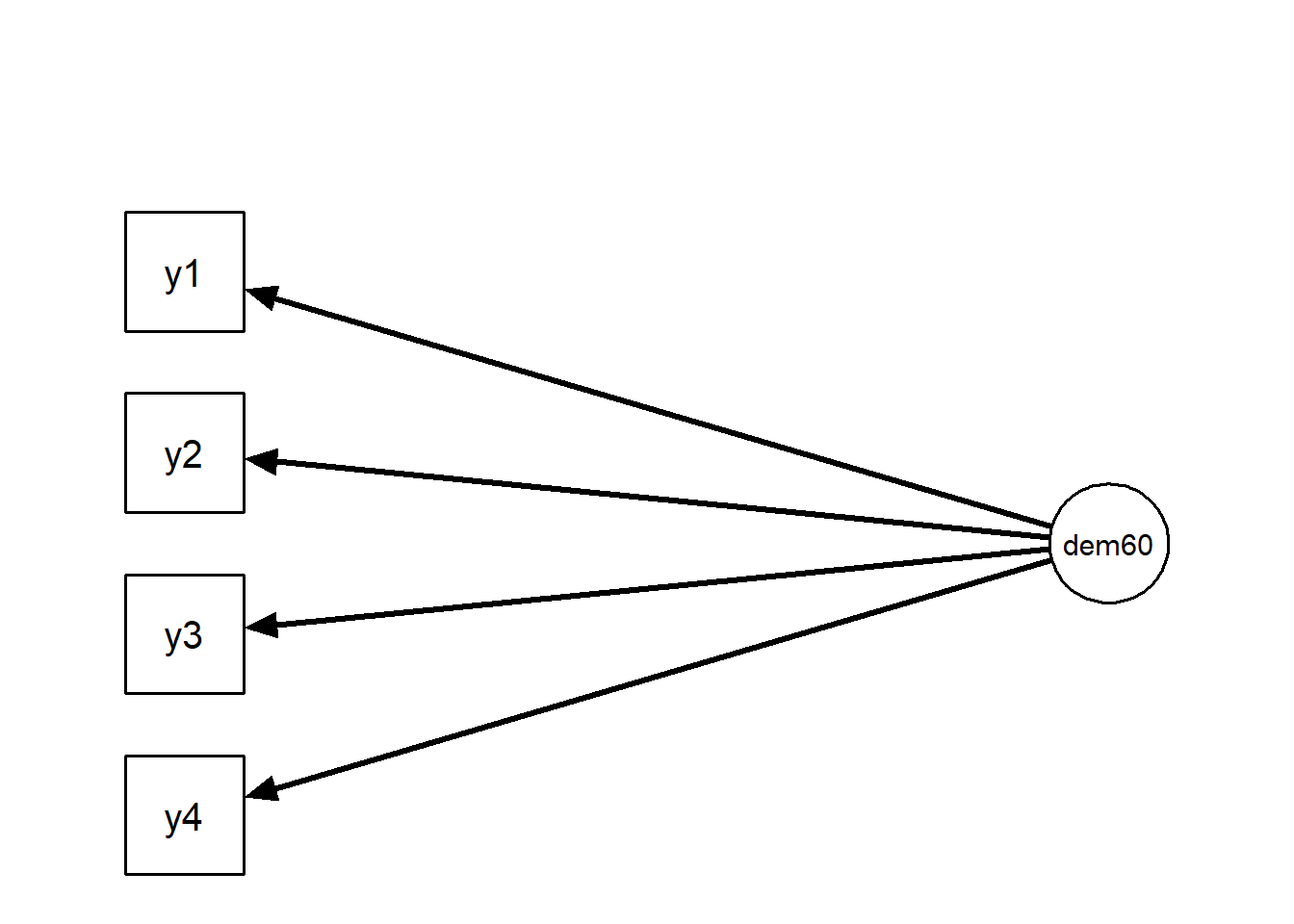Where

y$$_1$$ = Expert ratings of the freedom of the press in 1960

y$$_2$$ = The freedom of political opposition in 1960

y$$_3$$ = The fairness of elections in 1960

y$$_4$$ = The effectiveness of the elected legislature in 1960

dem60 = democracy in 1960

Note that dem60, the latent variable, is represented by a circle while y1-y4, the observed variables, are represented by squares. We are hypothesizing a model where the 4 observed variables are a function of the latent. Observed variables are also called indicators and latent variables are also called factors. The convention is to use the Greek letter $$\lambda$$ for representing the regression slopes of the relationships between the observed and latent variables. They are also called $$\textbf{factor loadings}$$.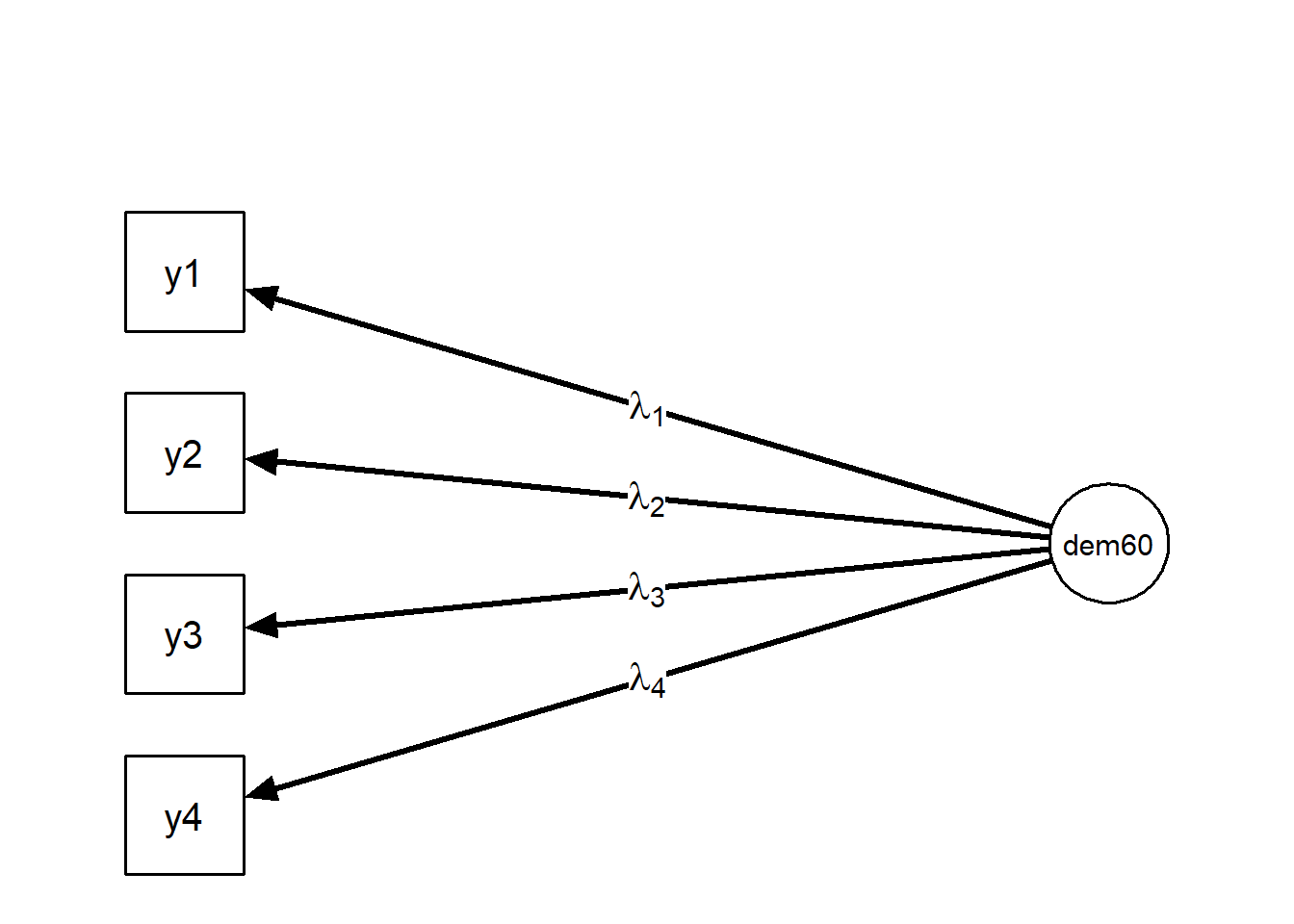The arrows point from the latent variable to the observed variables, indicating that we are hypothesizing a model in which the observed variables are a function of the latent. This means we suppose that the observed variables covary in such a way that we can assume a common cause for their variation (the latent variable). In order to test this model, we need to find the common variance of the indicators and test whether the latent variable can adequately explain iy. To find the common variance, we take a look at the variance-covariance matrix $$\textbf{S}$$ of our observed variables. Our aim is to compute, via a latent variable model, an implied variance-covariance matrix $$\mathbf{\Sigma}$$ that is similar to the observed $$\textbf{S}$$.

Before we start, let’s take a look at the parameters involved in computing the implied variance-covariance matrix.

• $$\textbf{Error}$$: The graph above looks neat, but reality never looks like this. There is always some noise in the data, and maybe we haven’t taken all relevant variables and/or covariances into account. First, we need to add an error term $$\varepsilon$$ to each of the equations: $$y_i = \lambda_i \cdot \eta_1 + \varepsilon_i$$

• $$\textbf{Residual variances}$$: this represents the variation of the error term. We will represent residual variances of the observed variables (i.e. that part of the variance that cannot be explained by the latent variables), using the Greek letter $$\theta$$ (in our case $$\theta_1$$, $$\theta_2$$, $$\theta_3$$, $$\theta_4$$).

• The latent variable has its own error term or $$\textbf{latent variance}$$, noted as $$\psi_{11}$$.

• $$\textbf{Intercepts}$$: this is the predicted value of the observed variable when the latent variable is zero. The intercepts have the same significance as in regression equations. In this case, the latent variable is the independent, and the observed variable is the dependent. Intercepts are noted with the Greek letter $$\tau$$. They are used to compare different groups or time points in measurement models.

• $$\textbf{Latent means}$$ are the means of the latent variables, noted with the Greek letter $$\alpha$$. These are usually set to 0, thus need not be estimated.

## Scaling

As the latent variables are not measured directly, we have no indication about their variance or mean, except for what we observe in the indicators. We need to $$\textbf{scale}$$ them in order to measure them. There are two ways of scaling: either by fixing the variance of the first latent variable in a multi-factor model, or by fixing the value of the first factor loading per each latent variable to 1. Psychonetrics automatically adopts the latter strategy, so you will not have to specify this in your model syntax.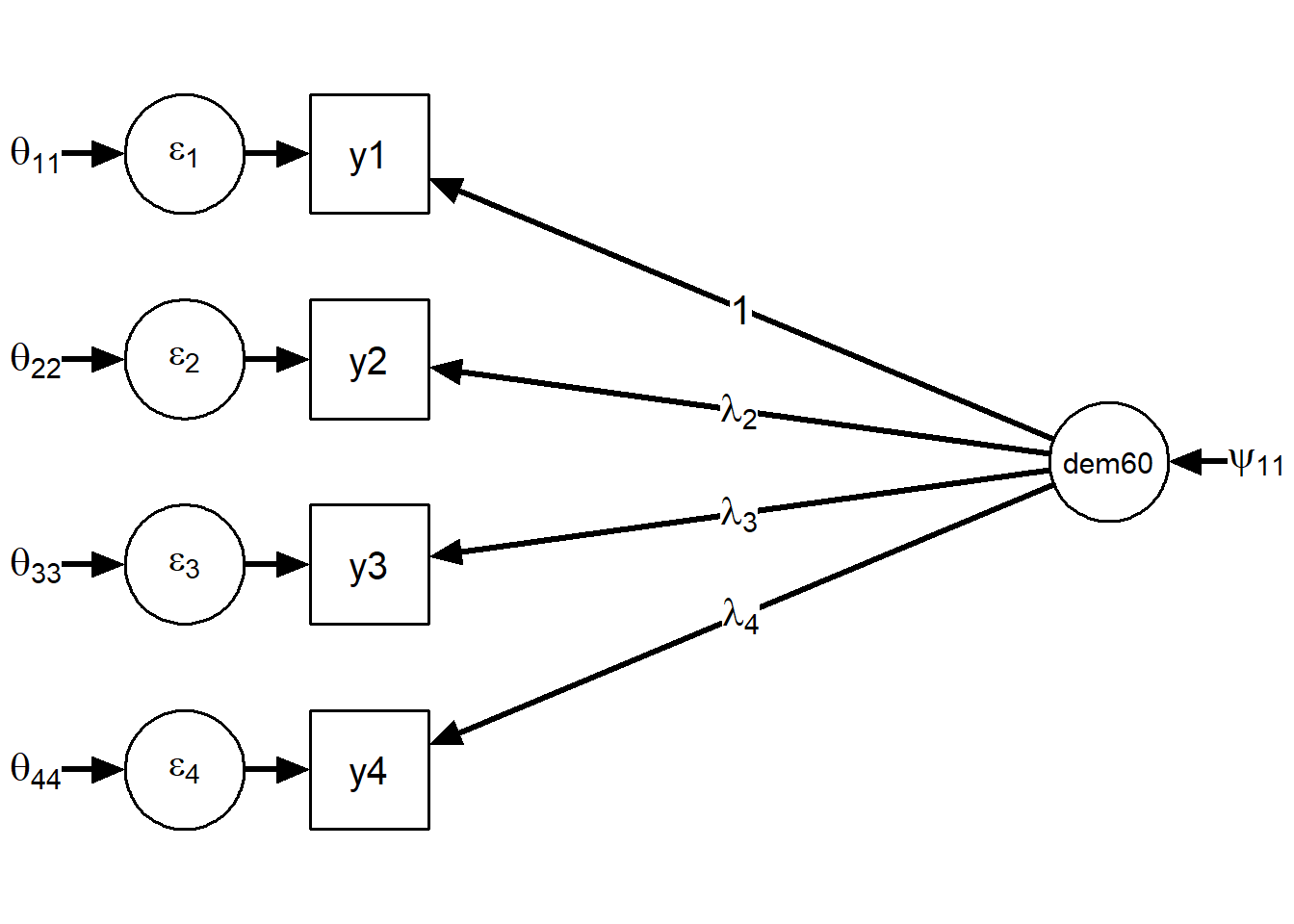Now we have the following:

• an observed variance-covariance matrix $$\textbf{S}$$ = $$\begin{bmatrix} var(y_1) & cov(y_1,y_2) & cov(y_1,y_3) & cov(y_1,y_4) \\ cov(y_2,y_1) & var(y_2) & cov(y_2,y_3) & cov(y_2,y_4) \\ cov(y_3,y_1) & cov(y_3,y_2) & var(y_3) & cov(y_3,y_4) \\ cov(y_4,y_1) & cov(y_4,y_2) & cov(y_4,y_3) & var(y_4) \end{bmatrix}$$ . $$\\*$$ $$\\*$$ Notice how this matrix is symmetric, i.e. elements over the diagonal “mirror” elements from under the diagonal. Actually, this matrix has $$\frac{n(n+1)}{2}$$ unique elements, in this case $$\frac{4(4+1)}{2} = 10$$ observed variances and covariances.

• a vector with the observed means $$\mathbf{\mu}$$ = $$\begin{bmatrix} \mu_1 \\ \mu_2 \\ \mu_3 \\ \mu_4 \end{bmatrix}$$

• a matrix $$\mathbf{\Lambda}$$ of factor loadings that we need to estimate: $$\mathbf{\Lambda} = \begin{bmatrix} 1 \\ \lambda_2 \\ \lambda_3 \\ \lambda_4 \end{bmatrix}$$ .$$\\*$$

• a matrix $$\mathbf{\Theta}$$ of residual variances and covariances that we need to estimate. $$\mathbf{\Theta} = \begin{bmatrix} \theta_{11} & \theta_{12} & \theta_{13} & \theta_{14} \\ \theta_{21} & \theta_{22} & \theta_{23} & \theta_{24} \\ \theta_{31} & \theta_{32} & \theta_{33} & \theta_{34} \\ \theta_{41} & \theta_{42} & \theta_{43} & \theta_{44} \\ \end{bmatrix}$$ $$\\*$$ As we only hypothesised residual variances, and no covariances, $$\mathbf{\Theta}$$ will be a diagonal matrix: $$\\*$$ $$\mathbf{\Theta} = \begin{bmatrix} \theta_{11} & 0 & 0 & 0 \\ 0 & \theta_{22} & 0 & 0 \\ 0 & 0 & \theta_{33} & 0 \\ 0 & 0 & 0 & \theta_{44} \\ \end{bmatrix}$$ $$\\*$$ The 0s mean that we $$\textit{fixed}$$ the corresponding residual covariances to 0, so they will not be estimated, but considered “known”.

• a matrix $$\mathbf{\Psi}$$ of latent variances and covariances that we need to estimate (which in our case has just one element) $$\mathbf{\Psi} = (\psi_{11})$$

Based on the data we will estimate, we can compute the implied variance-covariance matrix $$\mathbf\Sigma$$ using the formula:

$$\mathbf{\Sigma = \Lambda\Psi\Lambda^T + \Theta}$$

Then, we compare $$\Sigma$$ to $$\textbf{S}$$. If they do not differ significantly, then the model is an adequate description of or data. Let’s see how we can do this in $$\textbf{psychonetrics}$$.

First, we load the PoliticalDemocracy dataset from lavaan, and keep only the relevant variables

library(psychonetrics)
library(lavaan)
library(dplyr)

data <- PoliticalDemocracy %>%
select(y1:y4)

Now let’s test this model in $$\textbf{psychonetrics}$$. To do this correctly, we must outline the matrices conveying the relationship between the variables using specific syntax. The matrices will outline both the relations and the variables. We run a confirmatory factor analysis that will test the model that we built until now. In order to do this, we just tell $$\textbf{psychonetrics}$$ what our data is and how the $$\Lambda$$ matrix should look like. Then we use the $$\textbf{lvm()}$$ (latent variables model) function to build the model and we run it with $$\textbf{runmodel()}$$.

# Lambda matrix:
Lambda_dem60 <- matrix(c(
1, # dem60 =~ y1
1, # dem60 =~ y2
1, # dem60 =~ y3
1 # dem60 =~ y4
),ncol=1,byrow=TRUE)

cfa_dem <- lvm(
data,
lambda = Lambda_dem60,
latents = c("dem60")
)

cfa_dem <- cfa_dem %>%
runmodel

Note that $$\textbf{psychonetrics}$$ automatically computes modification indices? We will come back to that. First let’s look at the fit of the model.

## Model identification and fit

You can simply run the object “cfa_dem” to get an overview of the model’s $$\chi^2$$ measure of fit, degrees of freedom, and number of included parameters. This first test is very general and just gives you an overview of how well the parameters overall fit the data.

cfa_dem
##                         _                      _        _
##                        | |                    | |      (_)
##    _ __  ___ _   _  ___| |__   ___  _ __   ___| |_ _ __ _  ___ ___
##   |  _ \/ __| | | |/ __|  _ \ / _ \|  _ \ / _ \ __|  __| |/ __/ __|
##   | |_) \__ \ |_| | (__| | | | (_) | | | |  __/ |_| |  | | (__\__ \
##   | .__/|___/\__, |\___|_| |_|\___/|_| |_|\___|\__|_|  |_|\___|___/
##   | |         __/ |
##   |_|        |___/
##
##
## General:
##  - psychonetrics version: 0.7.2
##  - Model last edited at: 2020-05-15 11:26:24
##
## Sample:
##  - Number of cases: 75
##  - Number of groups: 1
##  - Number of observed summary statistics: 14
##
## Model:
##  - Model used: Latent variable model (LVM)
##  - Submodel used: Structural equation model (SEM)
##  - Number of parameters: 12
##
## Estimation:
##  - Optimizer used: nlminb
##  - Estimator used: Maximum likelihood estimation (ML)
##  - Message: relative convergence (4)
##
## Fit:
##  - Model Fit Test Statistic: 10.01
##  - Degrees of freedom: 2
##  - p-value (Chi-square): 0.0067
##
## Tips:
##  - Use 'psychonetrics::compare' to compare psychonetrics models
##  - Use 'psychonetrics::fit' to inspect model fit
##  - Use 'psychonetrics::parameters' to inspect model parameters
##  - Use 'psychonetrics::MIs' to inspect modification indices

The number of parameters tells you the numerical values estimated in the model. In our model, the parameters we wish to estimate include the $$\lambda$$s (we have 4 in our model, or do we?), the $$\psi$$s (our model has just 1), the $$\theta$$s (4 in our model), and the $$\tau$$s (4 in our model). If you jumped ahead and added the values to see if they sum up to 13, then you might have noticed that the output says something different. This is because we had to scale our latent variable to identify our model, meaning we decided to fix one $$\lambda$$ value per latent variable to one. By setting a $$\lambda$$ value to one we also remove it from the set of parameters to be estimated and thus it no longer counts to our total parameters. This removes 1 $$\lambda$$ value from our estimated parameters. There is another parameter that we can ignore for now, as it is fixed to 0: one $$\alpha$$ value, i.e. the mean of the latent variable.

Another rule for identifying a model is that the number of unique known values derived from the observed variance-covariance matrix should be equal to or greater than the number of unknown parameters we wish to estimate. To check if this is the case, you can calculate or check the degrees of freedom in a model. For more information on overall model identification, read Confirmatory Factor Analysis for Applied Research by Timothy Brown (2015, p. 61).

The number of Degrees of Freedom is computed by subtracting the number of parameters to estimate from the number of observed values (i.e. unique values in the variance-covariance matrix and means of our observed variables).

If the degrees of freedom are 0, then the model is just-identified and will fit the data perfectly.

If the degrees of freedom are < 0, then the model is underidentified. It is not possible to continue the analysis and you should alter your model to obtain a degree of freedom of at least 0.

If the degrees of freedom are > 0, then the model is overidentified. This means that the model likely does not fit your data perfectly and you should further evaluate how well the model fits by checking goodness-of-fit indices.

The Model Fit Test Statistic is the result of the $$\chi^2$$ test which compared our model to a saturated one. A saturated model is a model which fits the data perfectly (it has 0 degrees of freedom). Thus, this statistic with its p-value tells you to what extent your model differs from this perfectly fitting saturated model. If the p-value is below 0.05 this means that your model and the saturated model differ significantly (for $$\alpha$$ = 0.05), and thereby implies that your model does not fit the data perfectly.

Due to the way in which it is built, the $$\chi^2$$-test will tend to be significant for large sample sizes even when the differences are very slight, and therefore close fit indices are also insightful.

Our model is overidentified, meaning that in addition to the chi-square analysis, we will run goodness of fit analyses to further evaluate our model.

## Fit indices

Let’s look at the fit of our model

cfa_dem %>% fit
##            Measure   Value
##               logl -704.14
##  unrestricted.logl -699.13
##      baseline.logl -778.73
##               nvar       4
##               nobs      14
##               npar      12
##                 df       2
##          objective   11.43
##              chisq   10.01
##             pvalue  0.0067
##     baseline.chisq  159.18
##        baseline.df       6
##    baseline.pvalue     ~ 0
##                nfi    0.94
##               pnfi    0.31
##                tli    0.84
##               nnfi    0.84
##                rfi    0.81
##                ifi    0.95
##                rni    0.95
##                cfi    0.95
##              rmsea    0.23
##     rmsea.ci.lower    0.10
##     rmsea.ci.upper    0.38
##       rmsea.pvalue   0.014
##             aic.ll 1432.28
##            aic.ll2 1437.31
##              aic.x    6.01
##             aic.x2   34.01
##                bic 1460.09
##               bic2 1422.26
##            ebic.25 1476.72
##             ebic.5 1493.36
##            ebic.75 1506.66
##              ebic1 1526.63

First, you can interpret the RMSEA value labeled above as rmsea.pvalue. This measures the amount of misfit your model has per degrees of freedom. The smaller this value, the better the fit. Always also look at the confidence intervals of this value. Here are some rough guidelines of thresholds you can use for interpretation:

• < .05 “very good fit” or “close fit”
• .05 - .08 “good fit” or “fair fit”
• .08 - .1 “mediocre fit” or “good”
• .10 “poor” or “unacceptable”

Next, you can interpret the incremental fit indices: NFI, TLI, RFI, IFI, RNI, CFI. These compare your model to the baseline model. The baseline model is a model in which the variables are completely unrelated. The higher the value, the better your model is as compared to the baseline model with regard to your data. Here are some rough thresholds you can use to interpret your model fit using these indices:

0.9 - 0.95 “acceptable fit”" $$>$$ 0.95 “good fit”

You can find a more detailed and referenced discussion on fit indices here: http://www.davidakenny.net/cm/fit.htm

Our model doesn’t look well, does it?

## Parameters

We should also take a look at the estimated values for our parameters. You can find these in the ‘est’ column of the followng output:

cfa_dem %>% parameters
##
##  Parameters for group fullsample
##  -  nu
##  var1 op var2  est   se        p row col par
##    y1 ~1      5.46 0.30 < 0.0001   1   1   1
##    y2 ~1      4.26 0.45 < 0.0001   2   1   2
##    y3 ~1      6.56 0.38 < 0.0001   3   1   3
##    y4 ~1      4.45 0.38 < 0.0001   4   1   4
##
##  -  lambda
##  var1 op  var2  est   se        p row col par
##    y1 ~= dem60    1                 1   1   0
##    y2 ~= dem60 1.40 0.20 < 0.0001   2   1   5
##    y3 ~= dem60 1.09 0.17 < 0.0001   3   1   6
##    y4 ~= dem60 1.37 0.17 < 0.0001   4   1   7
##
##  -  sigma_zeta (symmetric)
##   var1 op  var2  est   se        p row col par
##  dem60 ~~ dem60 4.55 1.11 < 0.0001   1   1   8
##
##  -  sigma_epsilon (symmetric)
##  var1 op var2  est   se        p row col par
##    y1 ~~   y1 2.24 0.51 < 0.0001   1   1   9
##    y2 ~~   y2 6.41 1.29 < 0.0001   2   2  10
##    y3 ~~   y3 5.23 0.99 < 0.0001   3   3  11
##    y4 ~~   y4 2.53 0.77  0.00095   4   4  12

Remember all those Greek letters? We have now obtained their values! Let’s put them in a nice format:

“Tau” is the matrix with the intercepts: $$\mathbf{\tau}$$ = $$\begin{bmatrix} 5.46\\ 4.26 \\ 6.56 \\ 4.45 \\ \end{bmatrix}$$

“Lambda” is the matrix with the factor loadings: $$\mathbf{\Lambda}$$ = $$\begin{bmatrix} 1 \\ 1.40 \\ 1.09 \\ 1.37 \\ \end{bmatrix}$$. Notice how the factor loading for y$$_1$$ is fixed to 1. That is why it is not counted as an estimated parameter in the “par” column.

The $$\pmb{\Psi}$$ matrix is called “sigma_zeta” ($$\pmb{\Sigma}_\zeta$$) in $$\textbf{psychonetrics}$$ in order to signal that it is a variance-covariance matrix, where $$\zeta$$ stands for the latent residual ($$\textbf{psychonetrics}$$ uses an “all-y”-notation, where there is no difference made between endogenous and exogenous variables). It only has one estimate, as we have just one latent variable. $$\pmb{\Psi}$$ = $$\pmb{\Sigma}_\zeta$$ = $$\begin{bmatrix} 4.55 \\ \end{bmatrix}$$

The last matrix that $$\textbf{psychonetrics}$$ computes is called “sigma_epsilon” ($$\pmb{\Sigma}_\varepsilon$$), in order to signal that it is a variance-covariance matrix of the residuals ($$\varepsilon$$s). Earlier we called it $$\pmb{\Theta}$$, and we will stick to that notation as well, as it is commonly used in the literature. As we didn’t allow for any residual covariances (i.e. we fixed all covariances between $$\varepsilon$$s to 0), this matrix will be a diagonal one: $$\pmb{\Theta}$$ = $$\pmb{\Sigma}_\varepsilon$$ = $$\begin{bmatrix} 2.24 & 0 & 0 & 0 \\ 0 & 6.41 & 0 & 0 \\ 0 & 0 & 5.23 & 0 \\ 0 & 0 & 0 & 2.53 \\ \end{bmatrix}$$

## Modification indices

Could we improve our model so that it better fits the data? Let’s look at the modification indices. These are tools that help you reduce the RMSEA. Basically, the package computes alternative models, where it adds parameters to the original model. It compares each new model to the original model and lets us know what changes to our model would yield significant improvement. For this, we call the $$\textbf{MIs()}$$ function.

cfa_dem %>% MIs
##
## Top 10 modification indices:
##
##  var1 op var2      est       mi    pmi   epc        matrix row col      group
##    y4 ~~   y2 0.000000     9.25 0.0024  3.15 sigma_epsilon   4   2 fullsample
##    y3 ~~   y1 0.000000     9.25 0.0024  1.78 sigma_epsilon   3   1 fullsample
##    y4 ~~   y1 0.000000     5.34  0.021 -1.80 sigma_epsilon   4   1 fullsample
##    y3 ~~   y2 0.000000     5.34  0.021 -2.01 sigma_epsilon   3   2 fullsample
##    y4 ~~   y3 0.000000     0.70   0.40 -0.66 sigma_epsilon   4   3 fullsample
##    y2 ~~   y1 0.000000     0.70   0.40 -0.62 sigma_epsilon   2   1 fullsample
##    y2 ~~   y2 6.412377 < 0.0001    1.0   ~ 0 sigma_epsilon   2   2 fullsample
##    y3 ~~   y3 5.229089 < 0.0001    1.0   ~ 0 sigma_epsilon   3   3 fullsample
##    y1 ~1 <NA> 5.464667 < 0.0001      1   ~ 0            nu   1   1 fullsample
##    y2 ~1 <NA> 4.256443 < 0.0001      1   ~ 0            nu   2   1 fullsample
##  group_id
##         1
##         1
##         1
##         1
##         1
##         1
##         1
##         1
##         1
##         1

$$\textbf{psychonetrics}$$ orders the modification indices descendingly, those with the highest value (look at the “mi” column) will most improve the fit. Thus, the first two relations, $$y_4$$~~$$y_2$$ and $$y_3$$~ $$y_1$$ seem to be the most important ones. But do they make sense? Let’s look again at what the variables are:

y$$_1$$ = Expert ratings of the freedom of the press in 1960

y$$_2$$ = The freedom of political opposition in 1960

y$$_3$$ = The fairness of elections in 1960

y$$_4$$ = The effectiveness of the elected legislature in 1960

## Freeing parameters

It makes sense that the freedom of political opposition covaries with the effectiveness of the elected legislature, beyond what is explained by the variance of the latent variable state of democracy. So let’s “free” this parameter. This means that it won’t be fixed to 0 anymore, but will be freely estimated by $$\textbf{psychonetrics}$$. We do this with the aid of the function $$\textbf{freepar()}$$. Here we need to specify the matrix of the parameter and the location of the parameter within that matrix. Then we run the modified model.

cfa_dem_free <- cfa_dem %>%
freepar("sigma_epsilon", "y2", "y4") %>%
runmodel()

Let’s look at the fit of the new model.

cfa_dem_free %>% fit
##            Measure   Value
##               logl -699.97
##  unrestricted.logl -699.13
##      baseline.logl -778.73
##               nvar       4
##               nobs      14
##               npar      13
##                 df       1
##          objective   11.31
##              chisq    1.66
##             pvalue    0.20
##     baseline.chisq  159.18
##        baseline.df       6
##    baseline.pvalue     ~ 0
##                nfi    0.99
##               pnfi    0.16
##                tli    0.97
##               nnfi    0.97
##                rfi    0.94
##                ifi     1.0
##                rni     1.0
##                cfi     1.0
##              rmsea   0.094
##     rmsea.ci.lower     ~ 0
##     rmsea.ci.upper    0.34
##       rmsea.pvalue    0.24
##             aic.ll 1425.93
##            aic.ll2 1431.90
##              aic.x   -0.34
##             aic.x2   27.66
##                bic 1456.06
##               bic2 1415.09
##            ebic.25 1474.08
##             ebic.5 1492.10
##            ebic.75 1506.52
##              ebic1 1528.15

This looks already much better, even though RMSEA is not ideal. Now let’s look at the estimated parameters.

cfa_dem_free %>% parameters()
##
##  Parameters for group fullsample
##  -  nu
##  var1 op var2  est   se        p row col par
##    y1 ~1      5.46 0.30 < 0.0001   1   1   1
##    y2 ~1      4.26 0.45 < 0.0001   2   1   2
##    y3 ~1      6.56 0.38 < 0.0001   3   1   3
##    y4 ~1      4.45 0.38 < 0.0001   4   1   4
##
##  -  lambda
##  var1 op  var2  est   se        p row col par
##    y1 ~= dem60    1                 1   1   0
##    y2 ~= dem60 1.10 0.20 < 0.0001   2   1   5
##    y3 ~= dem60 1.06 0.15 < 0.0001   3   1   6
##    y4 ~= dem60 1.12 0.16 < 0.0001   4   1   7
##
##  -  sigma_zeta (symmetric)
##   var1 op  var2  est   se        p row col par
##  dem60 ~~ dem60 5.41 1.22 < 0.0001   1   1   8
##
##  -  sigma_epsilon (symmetric)
##  var1 op var2  est   se        p row col par
##    y1 ~~   y1 1.38 0.59    0.020   1   1   9
##    y2 ~~   y2 8.78 1.68 < 0.0001   2   2  10
##    y4 ~~   y2 2.70 1.05    0.010   4   2  11
##    y3 ~~   y3 4.49 0.96 < 0.0001   3   3  12
##    y4 ~~   y4 4.29 0.99 < 0.0001   4   4  13

Notice that the “sigma_epsilon” (i.e. $$\pmb{\Sigma}_{\varepsilon}$$ or $$\pmb{\Theta}$$) matrix has one more parameter. That matrix now looks as follows: $$\pmb{\Theta}$$ = $$\pmb{\Sigma}_\varepsilon$$ = $$\begin{bmatrix} 1.38 & 0 & 0 & 0 \\ 0 & 8.78 & 0 & 2.70 \\ 0 & 0 & 4.49 & 0 \\ 0 & 2.70 & 0 & 4.29 \\ \end{bmatrix}$$

The other parameters also changed slightly.

If we add these parameters to the graph, our model will look as follows: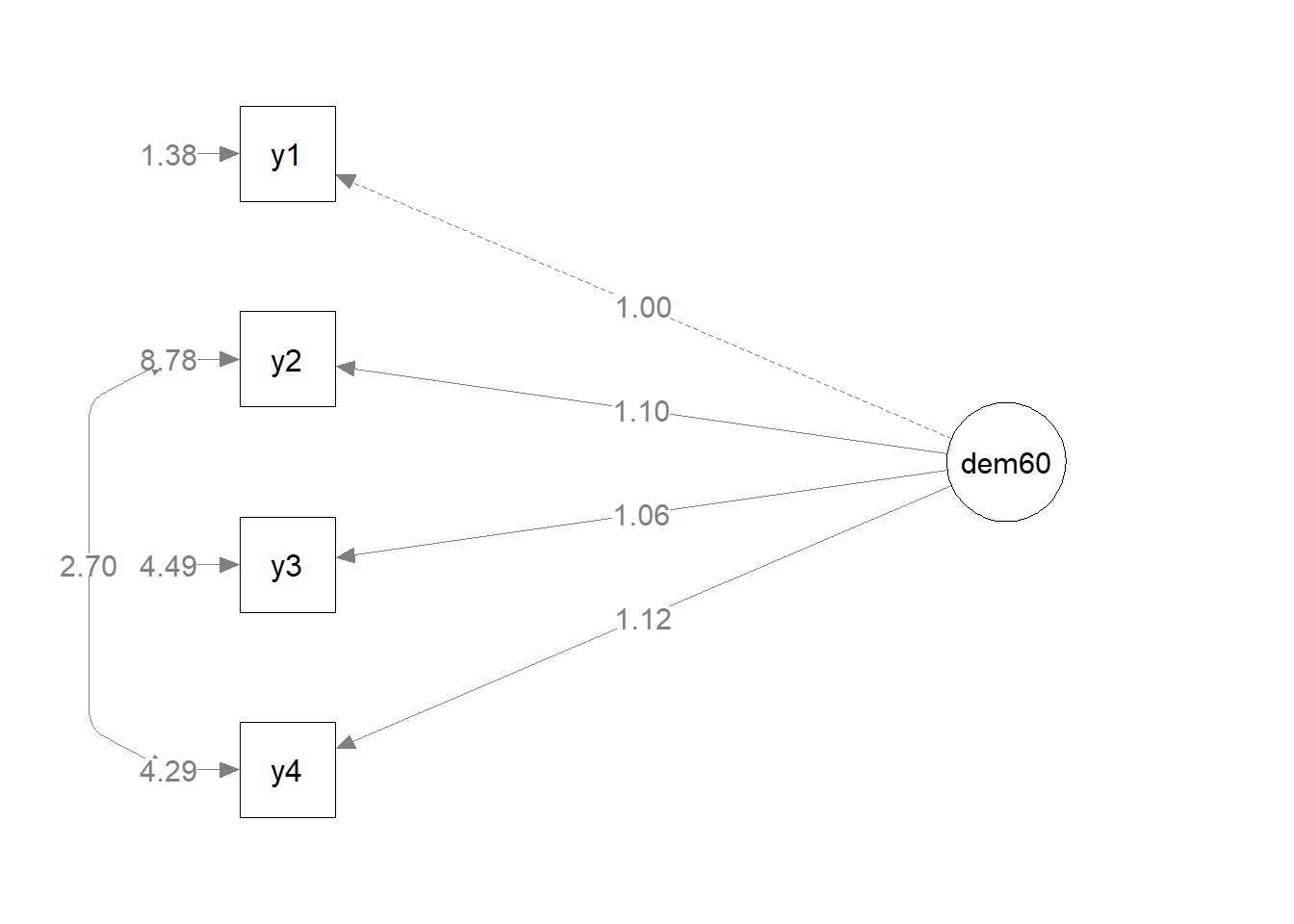Overall, our model seems to have improved considerably: RMSEA dropped from 0.23 (no fit) to 0.094 (mediocre fit). Also, $$\chi^2$$ is not significant anymore, meaning that the implied variance-covariance matrix $$\pmb{\Sigma}$$ does not differ significantly from the observed variance-covariance matrix $$\textbf{S}$$. This is great! Could we improve the model further? Let’s look at the modification indices again.

cfa_dem_free %>% MIs
##
## Top 10 modification indices:
##
##   var1 op  var2      est       mi  pmi   epc        matrix row col      group
##     y3 ~~    y2 0.000000     1.64 0.20 -1.01 sigma_epsilon   3   2 fullsample
##     y2 ~~    y1 0.000000     1.64 0.20  0.94 sigma_epsilon   2   1 fullsample
##     y4 ~~    y3 0.000000     1.64 0.20  1.02 sigma_epsilon   4   3 fullsample
##     y4 ~~    y1 0.000000     1.64 0.20 -0.96 sigma_epsilon   4   1 fullsample
##  dem60 ~~ dem60 5.410541 < 0.0001  1.0   ~ 0    sigma_zeta   1   1 fullsample
##     y1 ~1  <NA> 5.464667 < 0.0001    1   ~ 0            nu   1   1 fullsample
##     y2 ~1  <NA> 4.256443 < 0.0001    1   ~ 0            nu   2   1 fullsample
##     y3 ~1  <NA> 6.563110 < 0.0001    1   ~ 0            nu   3   1 fullsample
##     y4 ~1  <NA> 4.452533 < 0.0001    1   ~ 0            nu   4   1 fullsample
##  dem60 ~1  <NA> 0.000000 < 0.0001                   nu_eta   1   1 fullsample
##  group_id
##         1
##         1
##         1
##         1
##         1
##         1
##         1
##         1
##         1
##         1

There are no more significant modification indices (look at the “pmi” column), so we will keep our model as it is.

## Model Comparison

It is possible to directly compare models, but only if they are nested. This means that one of the models must be a special case of the other. Generally, the simpler model is nested within the modified one. Here, the covariance between y$$_4$$~~y$$_2$$ (or $$\theta_{42}$$ - equivalent to $$\theta_{24}$$ -, or $$\pmb{\Sigma}_\varepsilon$$$$_{42}$$ in $$\textbf{psychonetrics}$$ notation- equivalent to $$\pmb{\Sigma}_\varepsilon$$$$_{24}$$) in the modified model is estimated freely from the variance-covariance matrix (it could take any value, including 0), whereas in the simple model it is fixed to 0.

We noticed earlier that the second model, the one including the residual covariance y$$_4$$~~y$$_2$$, yielded better fit indicators than the original one. But is this model significantly better? The $$\textbf{compare()}$$ function from $$\textbf{psychonetrics}$$ lets us easily find out:

compare(Simple = cfa_dem, Modified = cfa_dem_free)

The $$\chi^2$$ is significant, meaining there is a significant difference between the models. We can check which model fits better by interpreting the AIC and BIC criteria. These are penalized-likelihood criteria which estimate how close the model is to a perfectly fitting model. The smaller the values are, the better the model fits the data.

Now, let’s add industrialization into the game. As stated above, this is another latent variable, that we measure with the help of three indicators:

x$$_1$$ = The gross national product (GNP) per capita in 1960

x$$_2$$ = The inanimate energy consumption per capita in 1960

x$$_3$$ = The percentage of the labor force in industry in 1960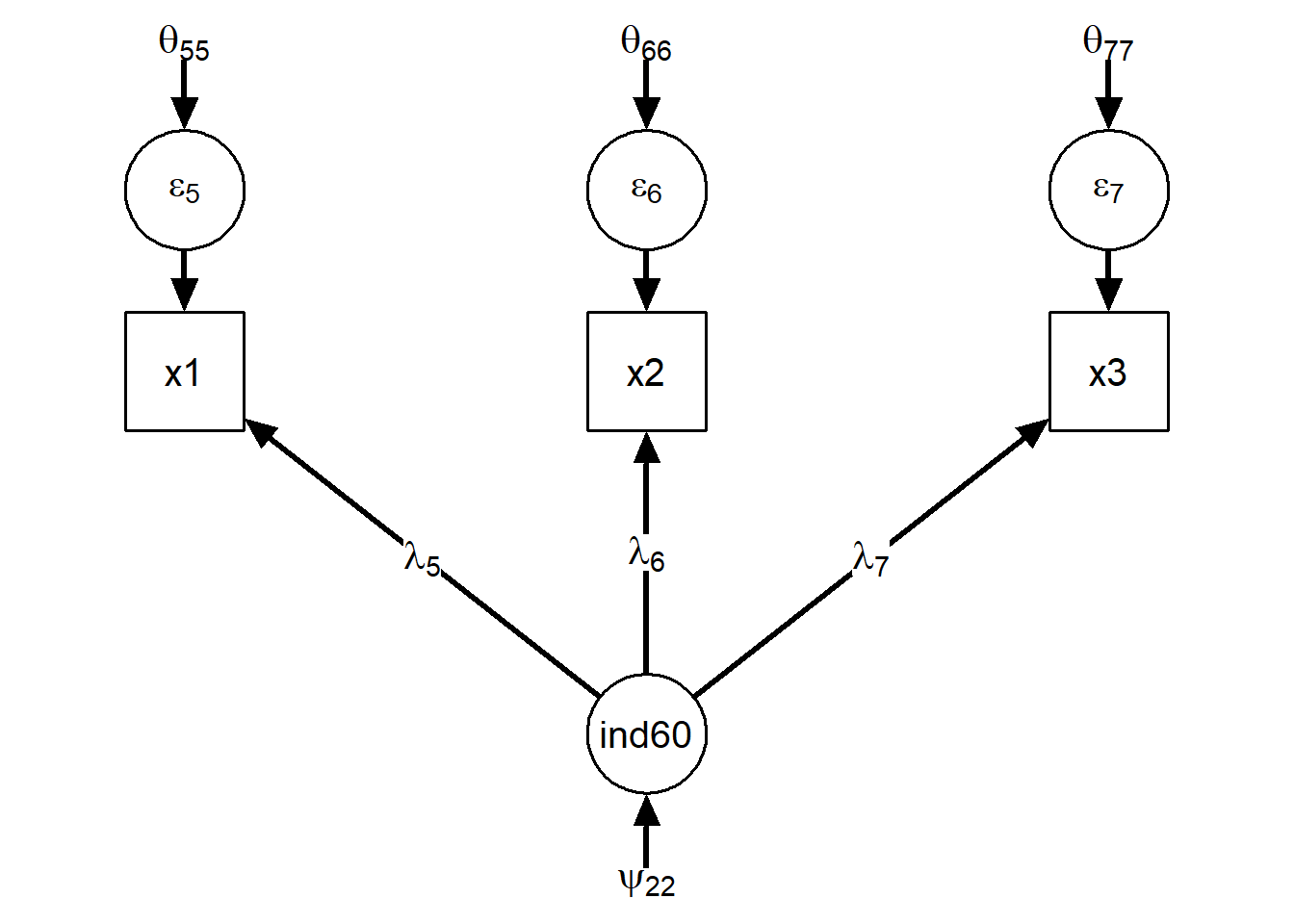Let’s run the confirmatory factor analysis for this model. We are hiding the chi-square output from this document via ‘suppressMessages’ to conserve space.

data <- PoliticalDemocracy %>%
select(x1:x3)

# Lambda matrix:
Lambda_ind60 <- matrix(c(
1, # ind60 =~ x1
1, # ind60 =~ x2
1 # ind60 =~ x3
),ncol=1,byrow=TRUE)

cfa_ind <- lvm(
data,
vars = paste0("x",1:3),
lambda = Lambda_ind60,
identification = "loadings",
latents = c("ind60")
)
cfa_ind <- cfa_ind %>%
runmodel

We can look at its fit, but keep in mind this is a saturated model (it has 0 degrees of freedom).

cfa_ind %>% fit
##            Measure   Value
##               logl -241.34
##  unrestricted.logl -241.34
##      baseline.logl -350.93
##               nvar       3
##               nobs       9
##               npar       9
##                 df     ~ 0
##          objective    0.92
##              chisq     ~ 0
##             pvalue     ~ 0
##     baseline.chisq  219.17
##        baseline.df       3
##    baseline.pvalue     ~ 0
##                nfi     1.0
##               pnfi     ~ 0
##                tli
##               nnfi       1
##                rfi
##                ifi     1.0
##                rni     1.0
##                cfi     1.0
##              rmsea
##     rmsea.ci.lower     ~ 0
##     rmsea.ci.upper     ~ 0
##       rmsea.pvalue     ~ 0
##             aic.ll  500.69
##            aic.ll2  503.46
##              aic.x     ~ 0
##             aic.x2   18.00
##                bic  521.55
##               bic2  493.18
##            ebic.25  531.43
##             ebic.5  541.32
##            ebic.75  549.23
##              ebic1  561.10

We can also ask $$\textbf{psychonetrics}$$ for the parameters.

cfa_ind %>% parameters

The graph for this model will look as follows: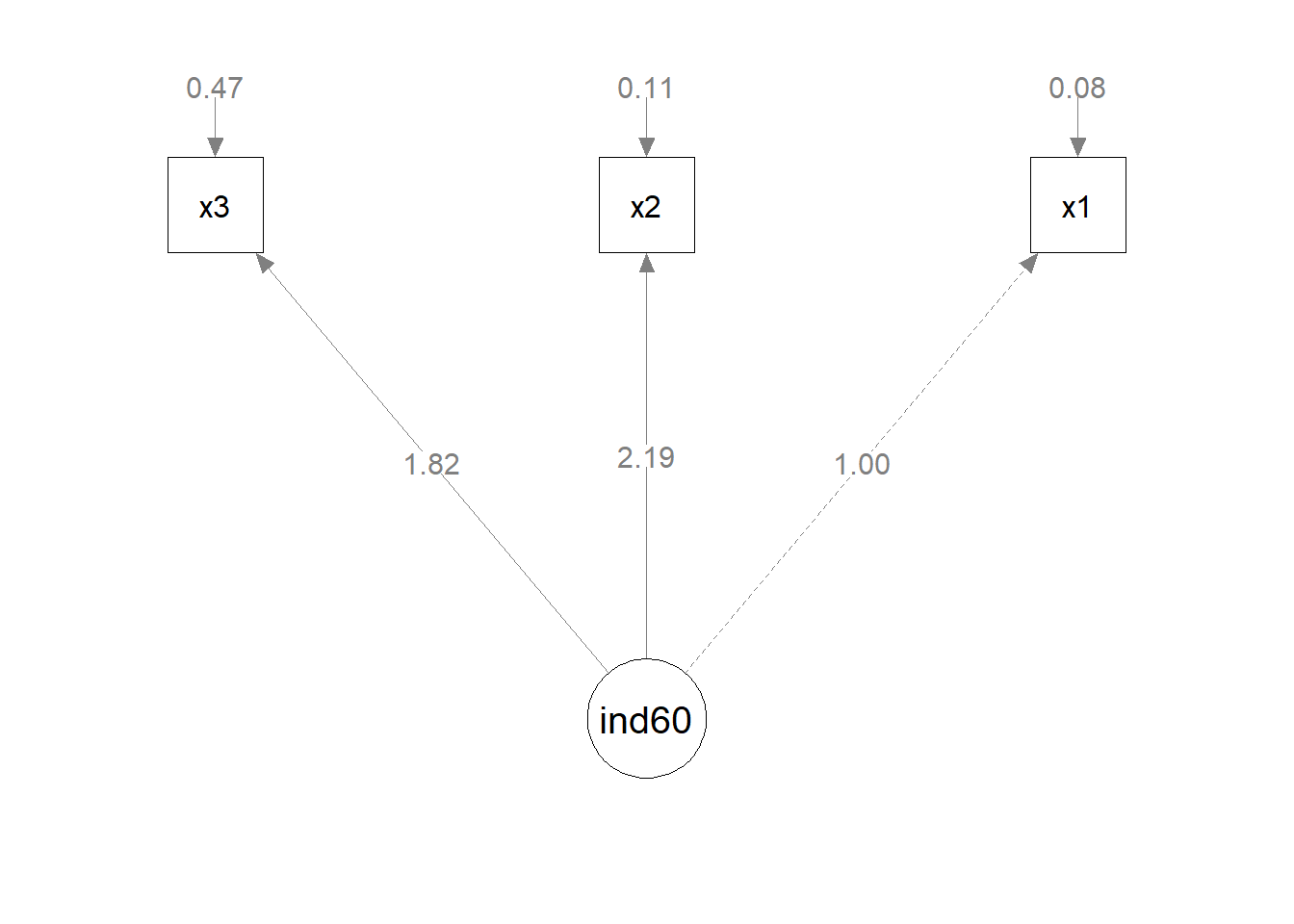# Structural Equations

Now let’s state the causal relationship between ind60 and dem60: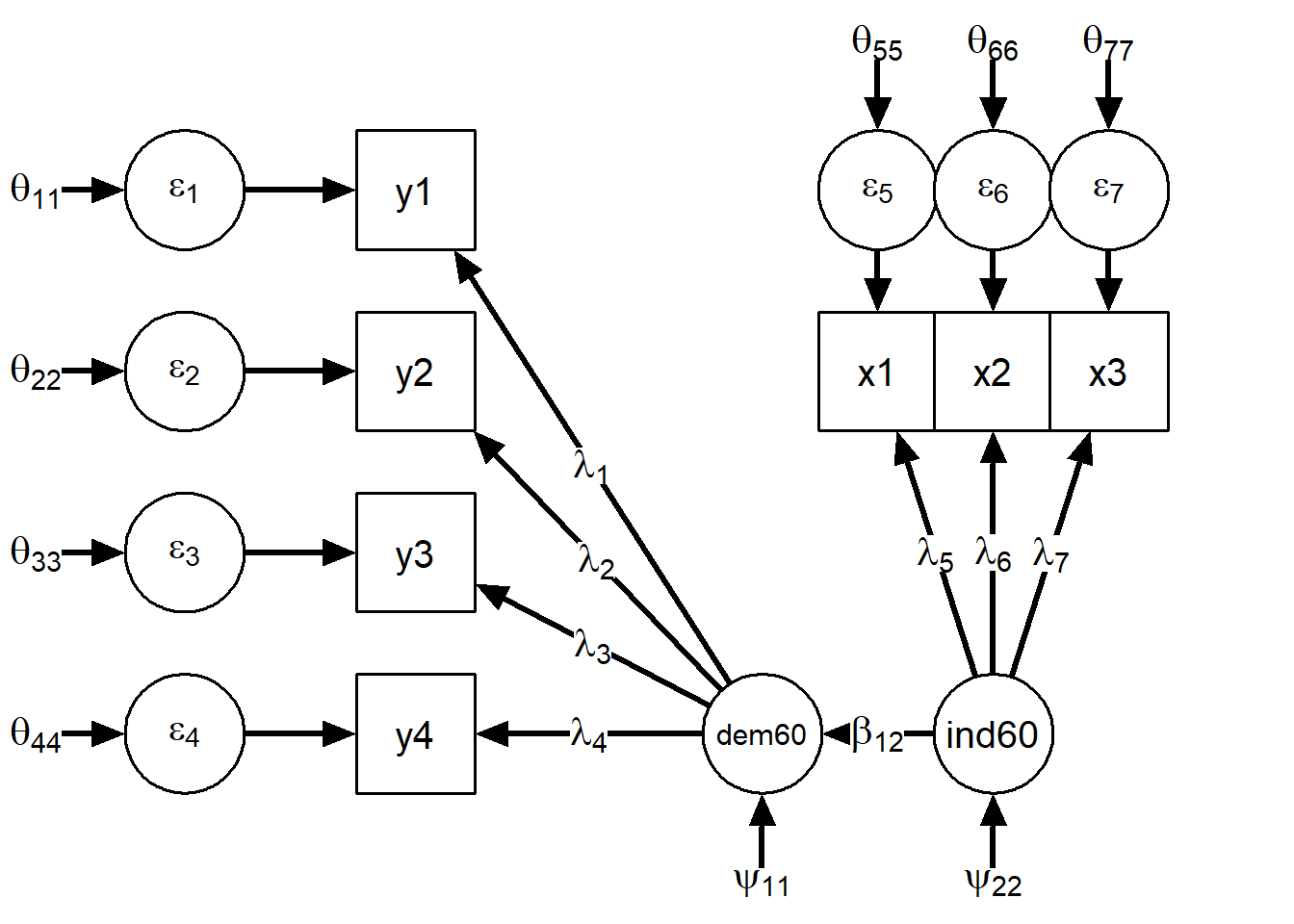Notice that a new Greek letter appeared: $$\beta$$, which is used to represent regression parameters. It indicates that we are introducing causal relations. It is only now that we are building a structural equation model. The previous two were measurement models, as they did not include causal relations between latents or between observed variables.

First, let’s build our dataset, with the indicators for democracy and industrialization in 1960.

data <- PoliticalDemocracy %>%
select(y1:y4, x1:x3)

Then, build the $$\Lambda$$ matrix (indicating where we expect to find non-zero factor loadings). Here the columns of the matrix represent the latent variables dem60 and ind60, respectively. The rows of the matrix represent the observed variables $$y_1-y_4$$ and $$x_1-x_3$$. A ‘1’ states that the observed variable in that row is related to the latent construct in that column while a ‘0’ states that the observed variable of that row is not related to the latent variable of that column.

# Lambda matrix:
Lambda_simple <- matrix(c(
1,0, # dem60 =~ y1
1,0, # dem60 =~ y2
1,0, # dem60 =~ y3
1,0, # dem60 =~ y4
0,1, # ind60 =~ x1
0,1, # ind60 =~ x2
0,1 # ind60 =~ x3
),ncol=2,byrow=TRUE)

and the $$\textbf{B}$$ matrix

# Beta matrix:
Beta_simple <- matrix(
c(0,1,
0,0),2,2,byrow=TRUE
)

Now let’s specify the model with the aid of the two matrices and run it.

# Model:
mod_simple <- lvm(data, lambda = Lambda_simple, beta = Beta_simple)

# Run model:
mod_simple <- mod_simple %>%
runmodel

Does our model fit?

# Look at fit:

mod_simple %>% fit
##            Measure    Value
##               logl  -938.08
##  unrestricted.logl  -926.21
##      baseline.logl -1129.65
##               nvar        7
##               nobs       35
##               npar       22
##                 df       13
##          objective    12.15
##              chisq    23.74
##             pvalue    0.034
##     baseline.chisq   406.88
##        baseline.df       21
##    baseline.pvalue      ~ 0
##                nfi     0.94
##               pnfi     0.58
##                tli     0.96
##               nnfi     0.96
##                rfi     0.91
##                ifi     0.97
##                rni     0.97
##                cfi     0.97
##              rmsea     0.10
##     rmsea.ci.lower    0.029
##     rmsea.ci.upper     0.17
##       rmsea.pvalue    0.093
##             aic.ll  1920.17
##            aic.ll2  1939.63
##              aic.x    -2.26
##             aic.x2    67.74
##                bic  1971.15
##               bic2  1901.81
##            ebic.25  2013.96
##             ebic.5  2056.77
##            ebic.75  2091.02
##              ebic1  2142.39

We should also take a look at the parameters.

mod_simple %>% parameters
##
##  Parameters for group fullsample
##  -  nu
##  var1 op var2  est    se        p row col par
##    y1 ~1      5.46  0.30 < 0.0001   1   1   1
##    y2 ~1      4.26  0.45 < 0.0001   2   1   2
##    y3 ~1      6.56  0.38 < 0.0001   3   1   3
##    y4 ~1      4.45  0.38 < 0.0001   4   1   4
##    x1 ~1      5.05 0.084 < 0.0001   5   1   5
##    x2 ~1      4.79  0.17 < 0.0001   6   1   6
##    x3 ~1      3.56  0.16 < 0.0001   7   1   7
##
##  -  lambda
##  var1 op  var2  est   se        p row col par
##    y1 ~= Eta_1    1                 1   1   0
##    y2 ~= Eta_1 1.42 0.20 < 0.0001   2   1   8
##    y3 ~= Eta_1 1.10 0.17 < 0.0001   3   1   9
##    y4 ~= Eta_1 1.43 0.17 < 0.0001   4   1  10
##    x1 ~= Eta_2    1                 5   2   0
##    x2 ~= Eta_2 2.18 0.14 < 0.0001   6   2  11
##    x3 ~= Eta_2 1.82 0.15 < 0.0001   7   2  12
##
##  -  beta
##   var1 op  var2  est   se       p row col par
##  Eta_1 <- Eta_2 1.44 0.38 0.00014   1   2  13
##
##  -  sigma_zeta (symmetric)
##   var1 op  var2  est    se        p row col par
##  Eta_1 ~~ Eta_1 3.45  0.87 < 0.0001   1   1  14
##  Eta_2 ~~ Eta_2 0.45 0.087 < 0.0001   2   2  15
##
##  -  sigma_epsilon (symmetric)
##  var1 op var2   est    se        p row col par
##    y1 ~~   y1  2.40  0.52 < 0.0001   1   1  16
##    y2 ~~   y2  6.55  1.29 < 0.0001   2   2  17
##    y3 ~~   y3  5.36   1.0 < 0.0001   3   3  18
##    y4 ~~   y4  2.14  0.72   0.0029   4   4  19
##    x1 ~~   x1 0.081 0.020 < 0.0001   5   5  20
##    x2 ~~   x2  0.12 0.072    0.094   6   6  21
##    x3 ~~   x3  0.47 0.091 < 0.0001   7   7  22

“Beta” is the matrix with the regression coefficients. “Eta1” ($$\eta_1$$) and “Eta2” ($$\eta_2$$) are the names $$\textbf{psychonetrics}$$ gave the latent variables, as it couldn’t guess that we are calling them “dem60” and “ind60”. We did not specify the names of the latent variables when we built the model. We can add them as follows, but this is optional. $$\textbf{psychonetrics}$$ can do without the names.

mod_simple <- lvm(data, lambda = Lambda_simple, beta = Beta_simple, latents = c("dem60", "ind60"))

$$\mathbf{B}$$ = $$\begin{bmatrix} 0 & 1.44 \\ 0 & 0 \\ \end{bmatrix}$$. There is only one $$\beta$$ parameter estimated, $$\beta_{12}$$ as we only hypothesised one causal relation: ind60 $$\rightarrow$$ dem60 (or, Eta2 $$\rightarrow$$ Eta1). Notice that the position in the matrix is given as such: the column has the number of the dependent, and the row has the number of the independent variable. All other possible $$\beta$$s were fixed to 0.

Our model doesn’t look very good yet, but we forgot something. We had to free the residual covariance between y$$_2$$ and y$$_4$$!

Our $$\pmb{\Theta}$$ ($$\pmb{\Sigma}_\varepsilon$$) matrix should look now as follows: $$\pmb{\Theta}$$ = $$\pmb{\Sigma}_\varepsilon$$ = $$\begin{bmatrix} \theta_{11} & . & . & . & . & . & .\\ 0 & \theta_{22} & . & . & . & . & .\\ 0 & 0 & \theta_{33} & . & . & . & .\\ 0 & \theta_{42} & 0 & \theta_{44} & . & . & .\\ 0 & 0 & 0 & 0 & \theta_{55} & . & .\\ 0 & 0 & 0 & 0 & 0 & \theta_{66} & .\\ 0 & 0 & 0 & 0 & 0 & 0 & \theta_{77} \end{bmatrix}$$

We now tell $$\textbf{psychonetrics}$$ to free the covariance between y$$_2$$ and y$$_4$$ and take a look at fit

mod_simple_modified <- mod_simple%>%
freepar("sigma_epsilon","y2","y4")  %>%
runmodel
mod_simple_modified %>% fit
##            Measure    Value
##               logl  -934.68
##  unrestricted.logl  -926.21
##      baseline.logl -1129.65
##               nvar        7
##               nobs       35
##               npar       23
##                 df       12
##          objective    12.06
##              chisq    16.94
##             pvalue     0.15
##     baseline.chisq   406.88
##        baseline.df       21
##    baseline.pvalue      ~ 0
##                nfi     0.96
##               pnfi     0.55
##                tli     0.98
##               nnfi     0.98
##                rfi     0.93
##                ifi     0.99
##                rni     0.99
##                cfi     0.99
##              rmsea    0.074
##     rmsea.ci.lower      ~ 0
##     rmsea.ci.upper     0.15
##       rmsea.pvalue     0.28
##             aic.ll  1915.36
##            aic.ll2  1937.01
##              aic.x    -7.06
##             aic.x2    62.94
##                bic  1968.67
##               bic2  1896.18
##            ebic.25  2013.42
##             ebic.5  2058.18
##            ebic.75  2093.98
##              ebic1  2147.69

This already looks much better! Remember that we can get the parameters by calling the $$\textbf{parameters}$$ function

mod_simple_modified %>% parameters

Our model now looks as follows: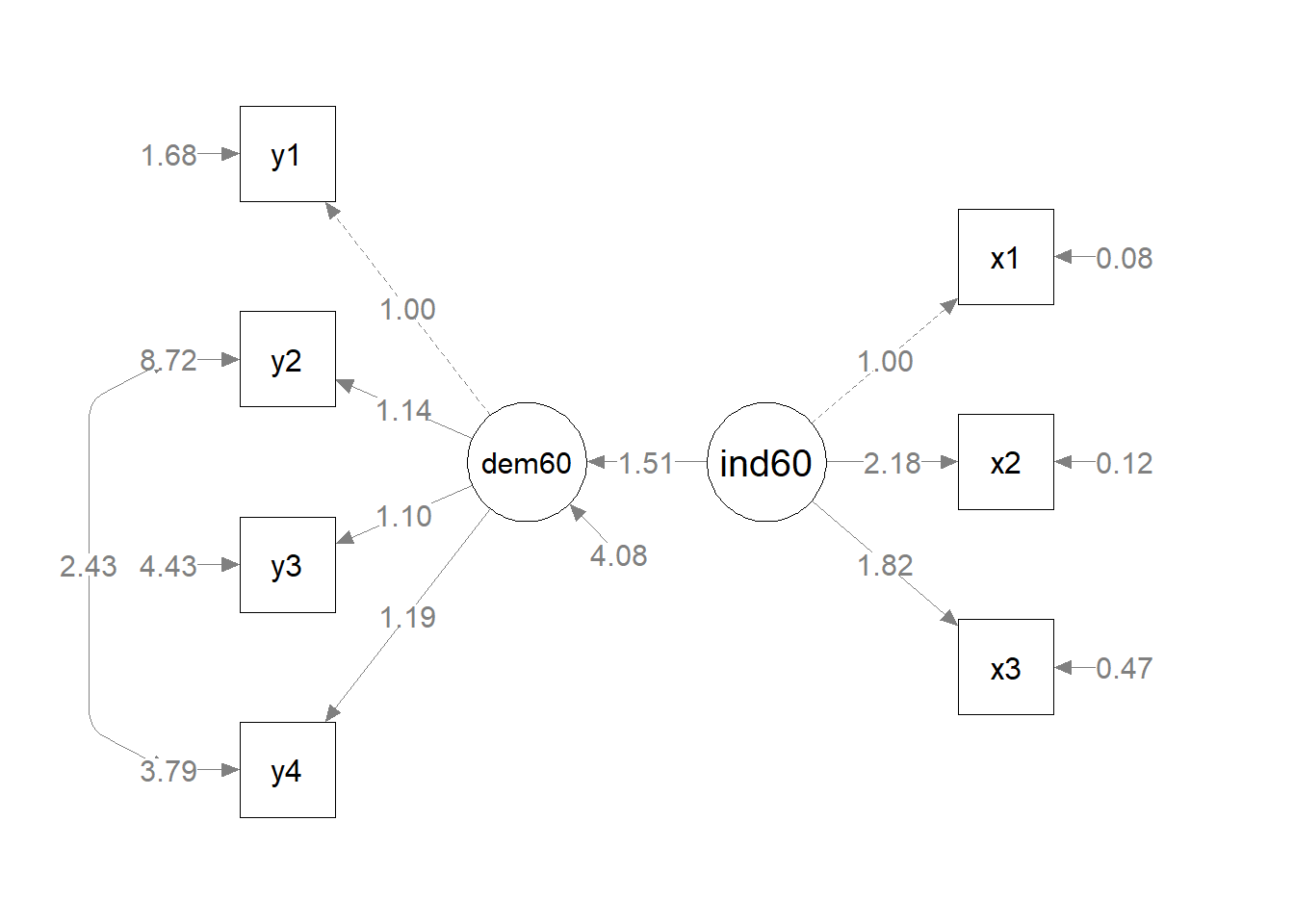# Full model

Let’s imagine that we can go from the year 1960 to 1965. In the meantime, we observe there have been some changes in the state of democracy (as observed through its indicators). We hypothesize that there is a direct effect of the state of democracy in 1960 on the state of democracy in 1965 (dem65). Also, we expect to find a direct effect of industrialization in 1960, in addition to the one mediated through dem60.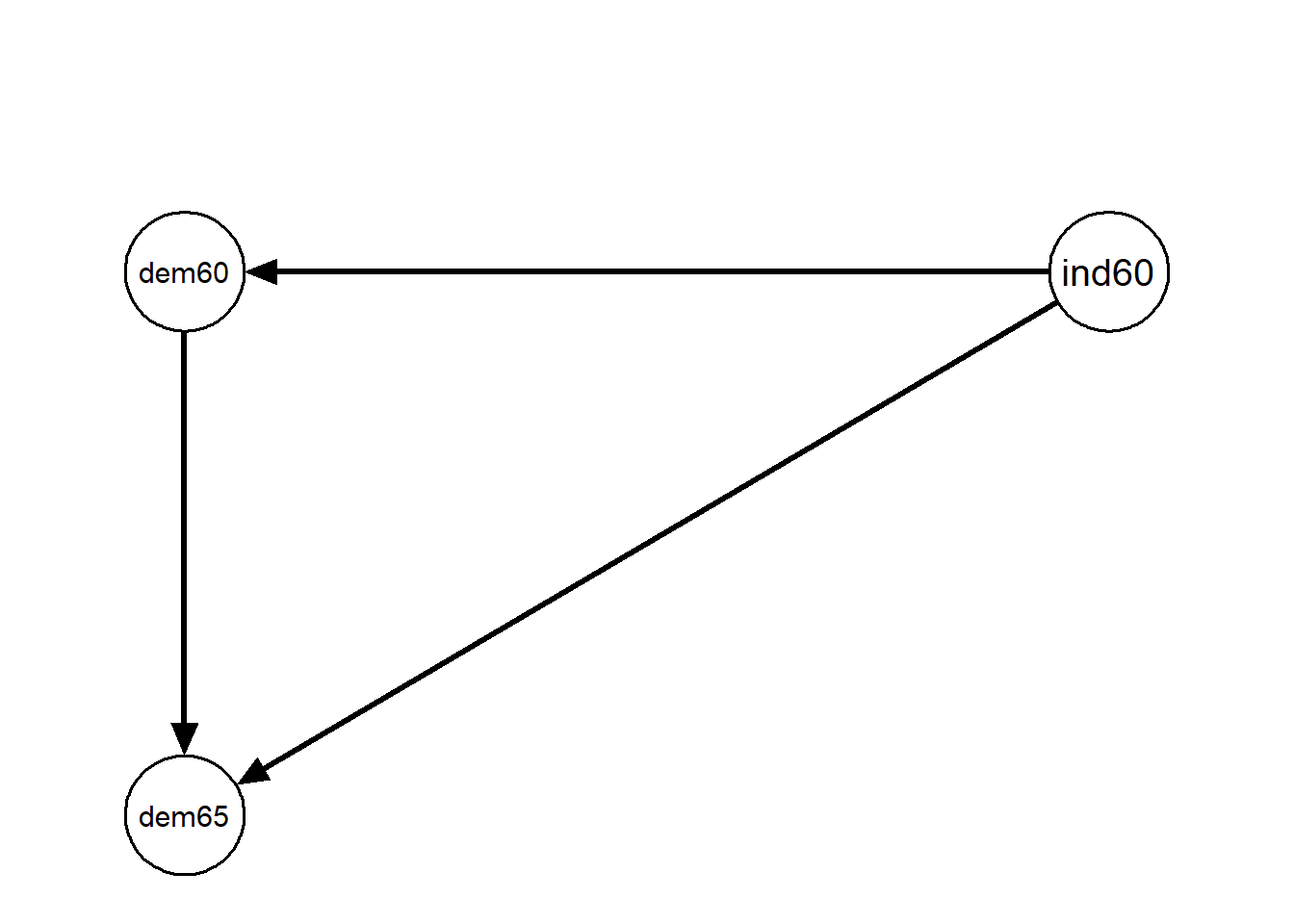It seems a bit bare, doesn’t it? Of course it does, because we are missing all the data! Let’s get our indicators into the model. For the state of democracy in 1965 we will use indicators similar to those collected in 1960, just with fresher data:

y$$_5$$ = Expert ratings of the freedom of the press in 1965

y$$_6$$ = The freedom of political opposition in 1965

y$$_7$$ = The fairness of elections in 1965

y$$_8$$ = The effectiveness of the elected legislature in 1965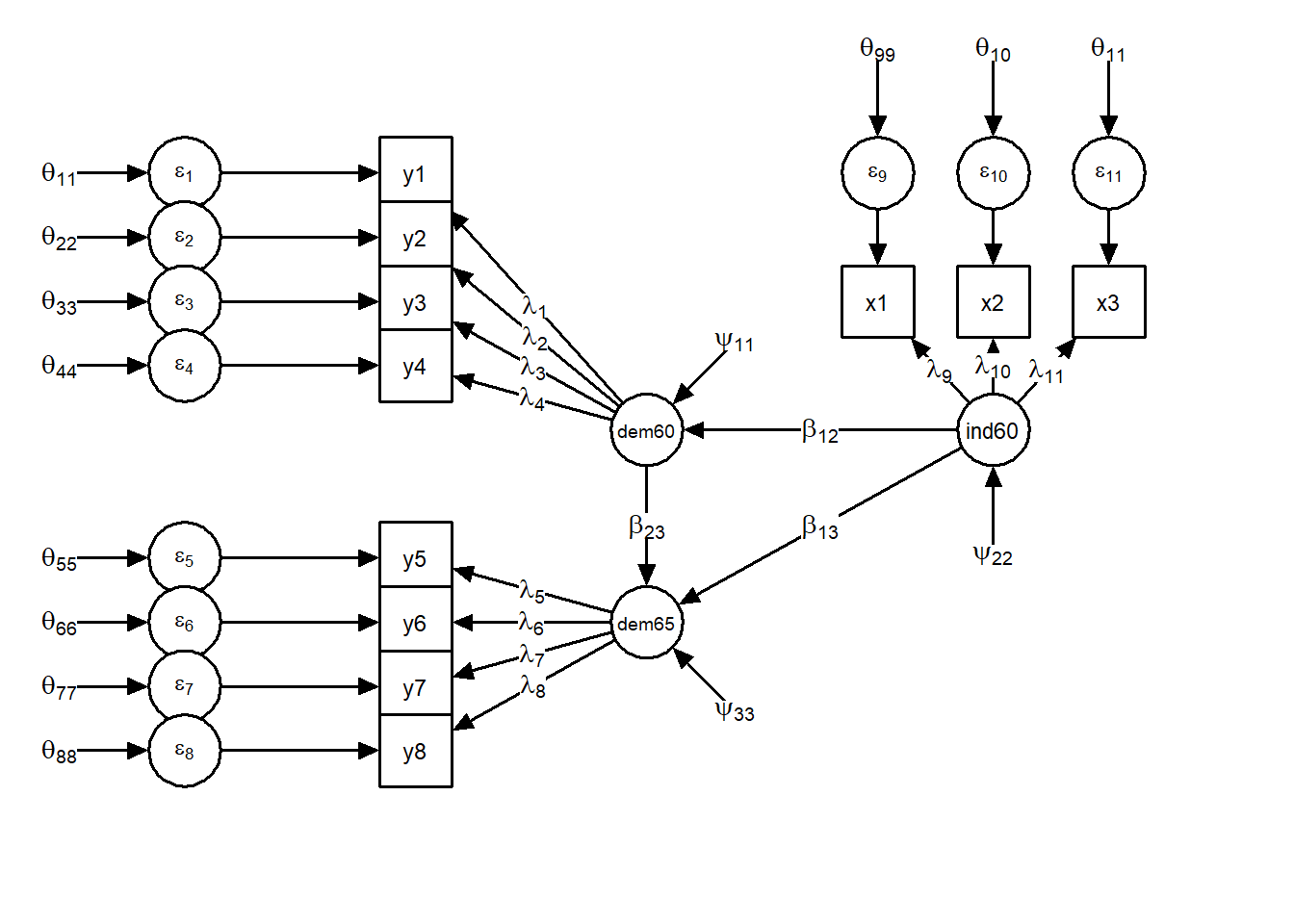Let’s test our model with $$\textbf{psychonetrics}$$. First, load the data:

data_full <- PoliticalDemocracy

And look at it

head(data_full)

The order of the variables is the right one (i.e. the one we need), so now we can proceed to build the $$\Lambda$$ matrix:

Lambda_full <- matrix(c(
1,0,0, # dem60 =~ y1
1,0,0, # dem60 =~ y2
1,0,0, # dem60 =~ y3
1,0,0, # dem60 =~ y4
0,1,0, # dem65 =~ y5
0,1,0, # dem65 =~ y6
0,1,0, # dem65 =~ y7
0,1,0, # dem65 =~ y8
0,0,1, # ind60 =~ x1
0,0,1, # ind60 =~ x2
0,0,1 # ind60 =~ x3
),ncol=3,byrow=TRUE)

And the $$\textbf{B}$$ matrix:

Beta_full <- matrix(
c(0,0,1,
1,0,1,
0,0,0),3,3,byrow=TRUE)

We can now build our model, run it, and assess its fit:

mod_full <- lvm(data_full, lambda = Lambda_full, beta = Beta_full) %>%
runmodel()

mod_full
##                         _                      _        _
##                        | |                    | |      (_)
##    _ __  ___ _   _  ___| |__   ___  _ __   ___| |_ _ __ _  ___ ___
##   |  _ \/ __| | | |/ __|  _ \ / _ \|  _ \ / _ \ __|  __| |/ __/ __|
##   | |_) \__ \ |_| | (__| | | | (_) | | | |  __/ |_| |  | | (__\__ \
##   | .__/|___/\__, |\___|_| |_|\___/|_| |_|\___|\__|_|  |_|\___|___/
##   | |         __/ |
##   |_|        |___/
##
##
## General:
##  - psychonetrics version: 0.7.2
##  - Model last edited at: 2020-05-15 11:26:28
##
## Sample:
##  - Number of cases: 75
##  - Number of groups: 1
##  - Number of observed summary statistics: 77
##
## Model:
##  - Model used: Latent variable model (LVM)
##  - Submodel used: Structural equation model (SEM)
##  - Number of parameters: 36
##
## Estimation:
##  - Optimizer used: nlminb
##  - Estimator used: Maximum likelihood estimation (ML)
##  - Message: relative convergence (4)
##
## Fit:
##  - Model Fit Test Statistic: 72.46
##  - Degrees of freedom: 41
##  - p-value (Chi-square): 0.0018
##
## Tips:
##  - Use 'psychonetrics::compare' to compare psychonetrics models
##  - Use 'psychonetrics::fit' to inspect model fit
##  - Use 'psychonetrics::parameters' to inspect model parameters
##  - Use 'psychonetrics::MIs' to inspect modification indices
mod_full %>% fit()
##            Measure    Value
##               logl -1564.96
##  unrestricted.logl -1528.73
##      baseline.logl -1894.06
##               nvar       11
##               nobs       77
##               npar       36
##                 df       41
##          objective    21.52
##              chisq    72.46
##             pvalue   0.0018
##     baseline.chisq   730.65
##        baseline.df       55
##    baseline.pvalue      ~ 0
##                nfi     0.90
##               pnfi     0.67
##                tli     0.94
##               nnfi     0.94
##                rfi     0.87
##                ifi     0.95
##                rni     0.95
##                cfi     0.95
##              rmsea     0.10
##     rmsea.ci.lower    0.061
##     rmsea.ci.upper     0.14
##       rmsea.pvalue    0.021
##             aic.ll  3201.92
##            aic.ll2  3272.02
##              aic.x    -9.54
##             aic.x2   144.46
##                bic  3285.35
##               bic2  3171.89
##            ebic.25  3371.67
##             ebic.5  3458.00
##            ebic.75  3527.06
##              ebic1  3630.64

This model doesn’t look too good… Haven’t we forgotten something? We needed to free the residual covariance between y$$_2$$ and y$$_4$$! Also, as the indicators for 1965 and 1960 are basically the same, we would expect a residual covariance between the corresponding variables for 1965: y$$_6$$ and y$$_8$$. Let’s free these parameters and see how the model fits.

mod_full_modified <- mod_full%>%
freepar("sigma_epsilon","y2","y4")  %>%
freepar("sigma_epsilon","y6","y8")  %>%
runmodel

mod_full_modified
##                         _                      _        _
##                        | |                    | |      (_)
##    _ __  ___ _   _  ___| |__   ___  _ __   ___| |_ _ __ _  ___ ___
##   |  _ \/ __| | | |/ __|  _ \ / _ \|  _ \ / _ \ __|  __| |/ __/ __|
##   | |_) \__ \ |_| | (__| | | | (_) | | | |  __/ |_| |  | | (__\__ \
##   | .__/|___/\__, |\___|_| |_|\___/|_| |_|\___|\__|_|  |_|\___|___/
##   | |         __/ |
##   |_|        |___/
##
##
## General:
##  - psychonetrics version: 0.7.2
##  - Model last edited at: 2020-05-15 11:26:28
##
## Sample:
##  - Number of cases: 75
##  - Number of groups: 1
##  - Number of observed summary statistics: 77
##
## Model:
##  - Model used: Latent variable model (LVM)
##  - Submodel used: Structural equation model (SEM)
##  - Number of parameters: 38
##
## Estimation:
##  - Optimizer used: nlminb
##  - Estimator used: Maximum likelihood estimation (ML)
##  - Message: relative convergence (4)
##
## Fit:
##  - Model Fit Test Statistic: 57.91
##  - Degrees of freedom: 39
##  - p-value (Chi-square): 0.026
##
## Tips:
##  - Use 'psychonetrics::compare' to compare psychonetrics models
##  - Use 'psychonetrics::fit' to inspect model fit
##  - Use 'psychonetrics::parameters' to inspect model parameters
##  - Use 'psychonetrics::MIs' to inspect modification indices
mod_full_modified %>% fit
##            Measure    Value
##               logl -1557.69
##  unrestricted.logl -1528.73
##      baseline.logl -1894.06
##               nvar       11
##               nobs       77
##               npar       38
##                 df       39
##          objective    21.32
##              chisq    57.91
##             pvalue    0.026
##     baseline.chisq   730.65
##        baseline.df       55
##    baseline.pvalue      ~ 0
##                nfi     0.92
##               pnfi     0.65
##                tli     0.96
##               nnfi     0.96
##                rfi     0.89
##                ifi     0.97
##                rni     0.97
##                cfi     0.97
##              rmsea    0.080
##     rmsea.ci.lower    0.029
##     rmsea.ci.upper     0.12
##       rmsea.pvalue     0.13
##             aic.ll  3191.37
##            aic.ll2  3273.70
##              aic.x   -20.09
##             aic.x2   133.91
##                bic  3279.44
##               bic2  3159.67
##            ebic.25  3370.56
##             ebic.5  3461.68
##            ebic.75  3534.57
##              ebic1  3643.92

We could still try to improve our model. As noted above, the variables y$$_5$$ - y$$_8$$ are basically a repeated measure of variables y$$_1$$ - y$$_4$$. We should therefore expect residual covariances between pairs of variables y$$_1$$ - y$$_5$$, y$$_2$$ - y$$_6$$ etc. Let’s run this model and look at the results.

mod_full_modified_history <- mod_full_modified%>%
freepar("sigma_epsilon","y1","y5")  %>%
freepar("sigma_epsilon","y2","y6")  %>%
freepar("sigma_epsilon","y3","y7")  %>%
freepar("sigma_epsilon","y4","y8")  %>%
runmodel

mod_full_modified_history %>% fit
##            Measure    Value
##               logl -1547.79
##  unrestricted.logl -1528.73
##      baseline.logl -1894.06
##               nvar       11
##               nobs       77
##               npar       42
##                 df       35
##          objective    21.06
##              chisq    38.13
##             pvalue     0.33
##     baseline.chisq   730.65
##        baseline.df       55
##    baseline.pvalue      ~ 0
##                nfi     0.95
##               pnfi     0.60
##                tli     0.99
##               nnfi     0.99
##                rfi     0.92
##                ifi      1.0
##                rni      1.0
##                cfi      1.0
##              rmsea    0.035
##     rmsea.ci.lower      ~ 0
##     rmsea.ci.upper    0.092
##       rmsea.pvalue     0.61
##             aic.ll  3179.58
##            aic.ll2  3292.46
##              aic.x   -31.87
##             aic.x2   122.13
##                bic  3276.92
##               bic2  3144.54
##            ebic.25  3377.63
##             ebic.5  3478.34
##            ebic.75  3558.91
##              ebic1  3679.76
mod_full_modified_history %>% parameters

Our model now looks as follows: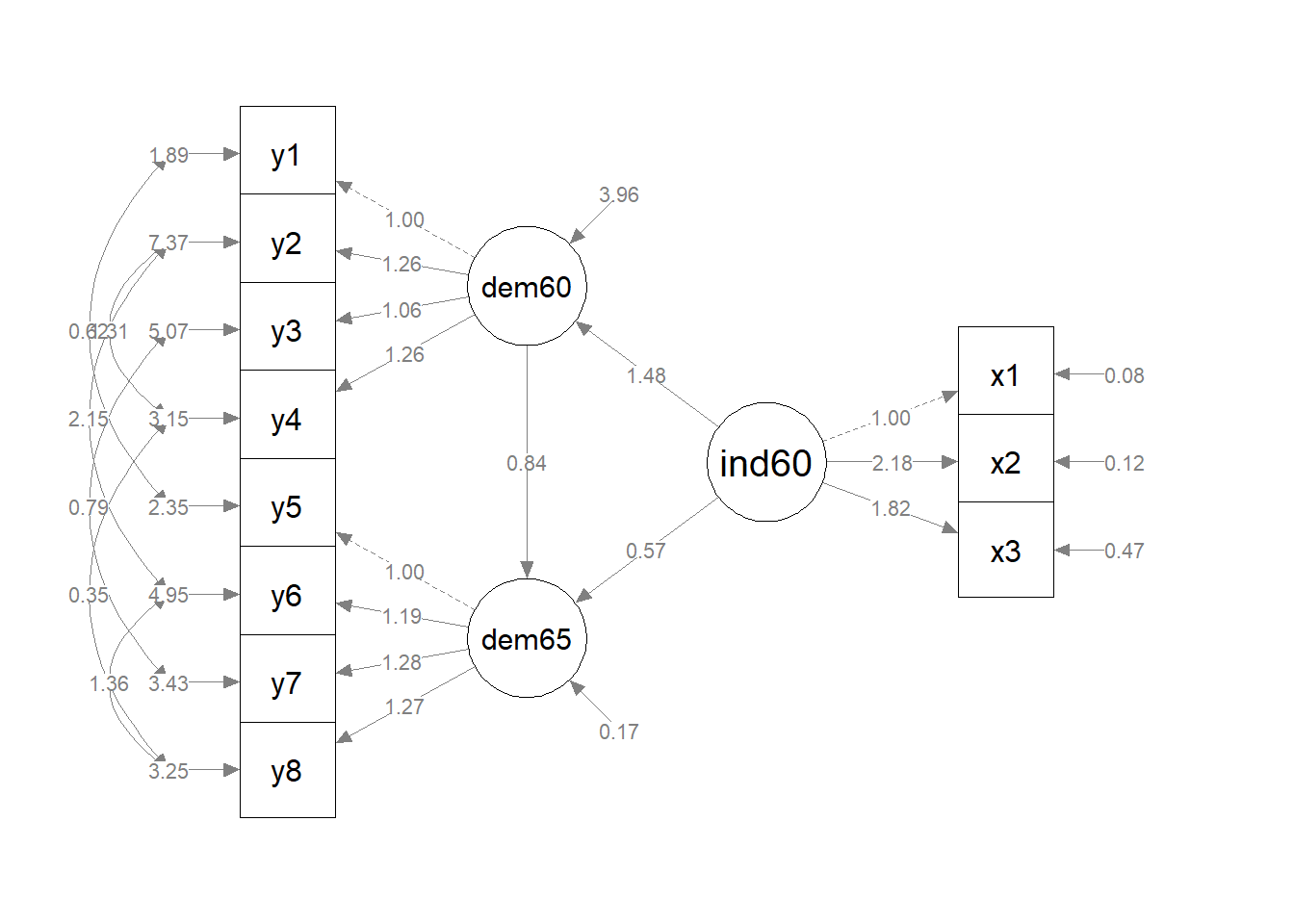## Fixing factor loadings accross latents variables

Suppose we assume that the relation between democracy and its indicators is stable. This would mean that the factor loadings of corresponding indicators would stay constant over time. We can fix them by giving them the same number in the $$\Lambda$$ matrix in $$\textbf{psychonetrics}$$. In this case, $$\textbf{psychonetrics}$$ will modify the equations so that the corresponding $$\lambda$$s are the same parameter to be estimated (notice the number of paramters in the output below!). The $$\textbf{B}$$ matrix will stay the same

Lambda_full_fixed <- matrix(c(
1,0,0, # dem60 =~ y1
2,0,0, # dem60 =~ y2
3,0,0, # dem60 =~ y3
4,0,0, # dem60 =~ y4
0,1,0, # dem65 =~ y5
0,2,0, # dem65 =~ y6
0,3,0, # dem65 =~ y7
0,4,0, # dem65 =~ y8
0,0,1, # ind60 =~ x1
0,0,1, # ind60 =~ x2
0,0,1 # ind60 =~ x3
),ncol=3,byrow=TRUE)

Beta_full <- matrix(
c(0,0,1,
1,0,1,
0,0,0),3,3,byrow=TRUE)

Don’t forget to free the parameters for residual covariances!

mod_full_fixed <- lvm(data_full, lambda = Lambda_full_fixed, beta = Beta_full) %>%
freepar("sigma_epsilon","y2","y4")  %>%
freepar("sigma_epsilon","y6","y8")  %>%
freepar("sigma_epsilon","y1","y5")  %>%
freepar("sigma_epsilon","y2","y6")  %>%
freepar("sigma_epsilon","y3","y7")  %>%
freepar("sigma_epsilon","y4","y8")  %>%
runmodel

An alternative way to do this is to use an input matrix for $$\pmb{\Sigma}_{\varepsilon}$$ (sigma_epsilon) with 1s for free parameters.

Sigma_epsilon = matrix(
c(1,0,0,0,1,0,0,0,0,0,0,
0,1,0,1,0,1,0,0,0,0,0,
0,0,1,0,0,0,1,0,0,0,0,
0,1,0,1,0,0,0,1,0,0,0,
1,0,0,0,1,0,0,0,0,0,0,
0,1,0,0,0,1,0,1,0,0,0,
0,0,1,0,0,0,1,0,0,0,0,
0,0,0,1,0,1,0,1,0,0,0,
0,0,0,0,0,0,0,0,1,0,0,
0,0,0,0,0,0,0,0,0,1,0,
0,0,0,0,0,0,0,0,0,0,1),11,11,byrow=TRUE)

mod_full_fixed <- lvm(data_full, lambda = Lambda_full_fixed, beta = Beta_full,
sigma_epsilon = Sigma_epsilon) %>%
runmodel

Let’s look at the fit

mod_full_fixed %>% fit
##            Measure    Value
##               logl -1548.82
##  unrestricted.logl -1528.73
##      baseline.logl -1894.06
##               nvar       11
##               nobs       77
##               npar       39
##                 df       38
##          objective    21.09
##              chisq    40.18
##             pvalue     0.37
##     baseline.chisq   730.65
##        baseline.df       55
##    baseline.pvalue      ~ 0
##                nfi     0.95
##               pnfi     0.65
##                tli      1.0
##               nnfi      1.0
##                rfi     0.92
##                ifi      1.0
##                rni      1.0
##                cfi      1.0
##              rmsea    0.028
##     rmsea.ci.lower      ~ 0
##     rmsea.ci.upper    0.087
##       rmsea.pvalue     0.67
##             aic.ll  3175.64
##            aic.ll2  3264.78
##              aic.x   -35.82
##             aic.x2   118.18
##                bic  3266.02
##               bic2  3143.10
##            ebic.25  3359.54
##             ebic.5  3453.05
##            ebic.75  3527.87
##              ebic1  3640.09

This looks really good!

And now the parameters:

mod_full_fixed %>% parameters

Our model now looks as follows: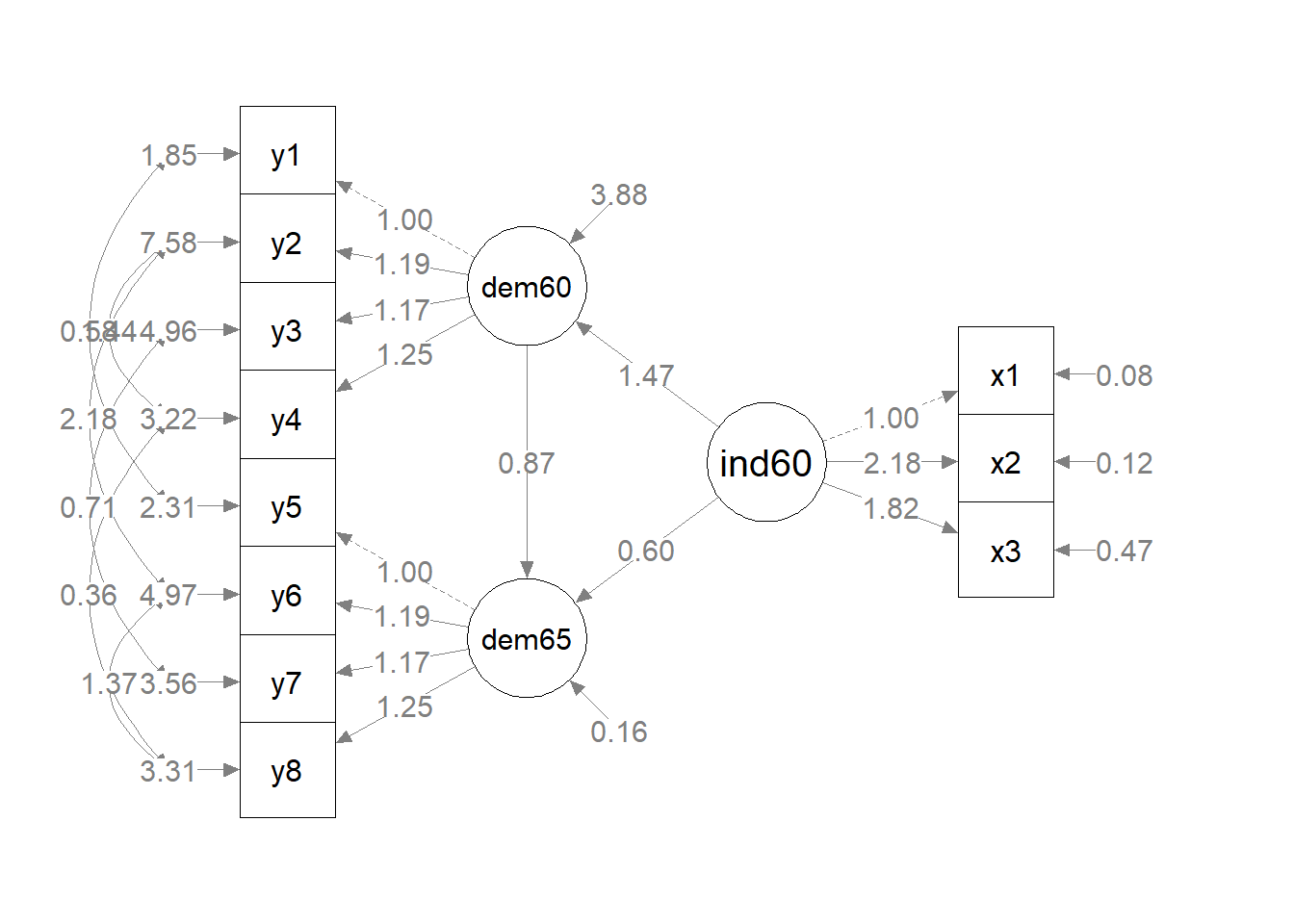# This was it, have fun!

With this tutorial you learned how to run confirmatory factor analyses and structural equation models in psychonetrics. You should now be able to specify and run models and interpret the outputs. You can use modification indices to improve your model, and you can compare nested models. $$\textbf{psychonetrics}$$ is still under development, and it will be expanded to include for example a function that improves the model automatically by freeing paramters ($$\textbf{stepup()}$$). Also, there should soon be a way of plotting the models built in $$\textbf{psychonetrics}$$.